# Maharashtra Board 10th Class Maths Part 2 Practice Set 7.1 Solutions Chapter 7 Mensuration

Balbharti Maharashtra State Board Class 10 Maths Solutions covers the Practice Set 7.1  Geometry 10th Class Maths Part 2 Answers Solutions Chapter 7 Mensuration.

## Practice Set 7.1 Geometry 10th Std Maths Part 2 Answers Chapter 7 Mensuration

Practice Set 7.1 Geometry 10th Question 1. Find the volume of a cone if the radius of its base is 1.5 cm and its perpendicular height is 5 cm.
Given: For the cone,
perpendicular height (h) = 5 cm
To find: Volume of the cone.
Solution:
Volume of cone = $$\frac { 1 }{ 3 }$$ πr2h∴ The volume of the cone is 11.79 cm3.

Mensuration Practice Set 7.1 Question 2. Find the volume of a sphere of diameter 6 cm. [π = 3.14]
Given: For the sphere, diameter (d) = 6 cm
To find: Volume of the sphere.
Solution:
Radius (r) = $$\frac { d }{ 2 }$$ = $$\frac { 6 }{ 2 }$$ = 3 cm
Volume of sphere = $$\frac { 4 }{ 3 }$$ πr2
= $$\frac { 4 }{ 3 }$$ × 3.14 × (3)3
= 4 × 3.14 × 3 × 3
= 113.04 cm3
∴ The volume of the sphere is 113.04 cm3.

Practice Set 7.1 Geometry Class 10 Question 3. Find the total surface area of a cylinder if the radius of its base is 5 cm and height is 40 cm. [π = 3.14]
Given: For the cylinder,
height (h) = 40 cm
To find: Total surface area of the cylinder.
Solution:
Total surface area of cylinder = 2πr (r + h)
= 2 × 3.14 × 5 (5 + 40)
= 2 × 3.14 × 5 × 45
= 1413 cm2
The total surface area of the cylinder is 1413 cm2.

Practice Set 7.1 Geometry Question 4. Find the surface area of a sphere of radius 7 cm.
Given: For the sphere, radius (r) = 7 cm
To find: Surface area of the sphere.
Solution:
Surface area of sphere = Aπr2
= 4 × $$\frac { 22 }{ 7 }$$ × (7)2
= 88 × 7
= 616 cm2
∴ The surface area of the sphere is 616 cm2.

Practice Set 7.1 Question 5. The dimensions of a cuboid are 44 cm, 21 cm, 12 cm. It is melted and a cone of height 24 cm is made. Find the radius of its base.
Given: For the cuboid,
length (l) = 44 cm, breadth (b) = 21 cm,
height (h) = 12 cm
For the cone, height (H) = 24 cm
To find: Radius of base of the cone (r).
Solution:
Volume of cuboid = l × b × h
= 44 × 21 × 12 cm3
Volume of cone = $$\frac { 1 }{ 3 }$$ πr2H
= $$\frac { 1 }{ 3 }$$ × $$\frac { 22 }{ 7 }$$ × r2 × 24 cm3
Since the cuboid is melted to form a cone,
∴ volume of cuboid = volume of cone∴ r2 = 21 × 21
∴ r = 21 cm …[Taking square root of both sides]
∴ The radius of the base of the cone is 21 cm.

10th Class Geometry Practice Set 7.1 Question 6. Observe the measures of pots in the given figures. How many jugs of water can the cylindrical pot hold?Given: For the conical water jug,
radius (r) = 3.5 cm, height (h) = 10 cm
For the cylindrical water pot,
radius (R) = 7 cm, height (H) = 10 cm
To find: Number of jugs of water the cylindrical pot can hold.
Solution:
Volume of conical jug = $$\frac { 1 }{ 3 }$$ πr2h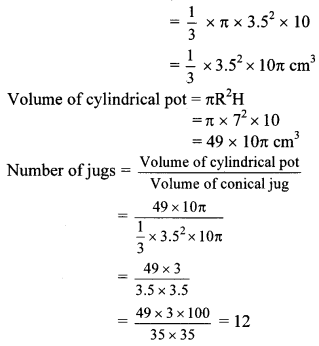∴ The cylindrical pot can hold 12 jugs of water.

Mensuration Class 10 Practice Set 7.1 Question 7. A cylinder and a cone have equal bases. The height of the cylinder is 3 cm and the area of its base is 100 cm2. The cone is placed up on the cylinder. Volume of the solid figure so formed is 500 cm3. Find the total height of the figureGiven: For the cylindrical part,
height (h) = 3 cm,
area of the base (πr2)= 100 cm2
Volume of the entire figure = 500 cm3
To find: Total height of the figure.
Solution:
A cylinder and a cone have equal bases.
Area of base = 100 cm2
∴ πr2 =100 …(i)
Let the height of the conical part be H.
Volume of the entire figure
= Volume of the entire + Volume of cone∴ Height of conical part (H) =6 cm
Total height of the figure = h + H
= 3 + 6
= 9 cm
∴ The total height of the figure is 9 cm.

10th Geometry Practice Set 7.1 Question 8. In the given figure, a toy made from a hemisphere, a cylinder and a cone is shown. Find the total area of the toy.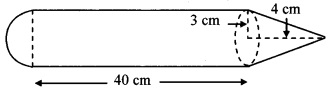Given: For the conical Part,
height (h) = 4 cm, radius (r) = 3 cm
For the cylindrical part,
height (H) = 40 cm, radius (r) = 3 cm
For the hemispherical part,
To find: Total area of the toy.
Solution:
Slant height of cone (l) = $$\sqrt{\mathrm{h}^{2}+\mathrm{r}^{2}}$$
= $$\sqrt{\mathrm{4}^{2}+\mathrm{3}^{2}}$$
= $$\sqrt { 16+9 }$$
= $$\sqrt { 25 }$$ = 5 cm
Curved surface area of cone = πrl
= π × 3 × 5
= 15π cm2
Curved surface area of cylinder = 2πrH
= 2 × π × 3 × 40
= 240π cm2
Curved surface area of hemisphere = 2πr2
= 2 × π × 32
= 18π cm2
Total area of the toy
= Curved surface area of cone + Curved surface area of cylinder + Curved surface area of hemisphere
= 15π + 240π + 18π
= 2737 π cm2
∴ The total area of the toy is 273π cm2.

7.1.8 Practice Question 9. In the given figure, a cylindrical wrapper of flat tablets is shown. The radius of a tablet is 7 mm and its thickness is 5 mm. How many such tablets are wrapped in the wrapper?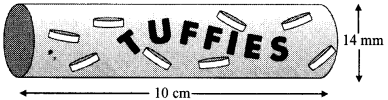Given: For the cylindrical tablets,
thickness = height(h) = 5 mm
For the cylindrical wrapper,
diameter (D) = 14 mm, height (H) = 10 cm
To find: Number of tablets that can be wrapped.
Solution:
Radius of wrapper (R) = $$\frac { Diameter }{ 2 }$$
= $$\frac { 14 }{ 2 }$$ = 7 mm
Height of wrapper (H) = 10 cm
= 10 × 10 mm
= 100 mm
Volume of a cylindrical wrapper = πR2H
= π(7)2 × 100
= 4900π mm3
Volume of a cylindrical tablet = πr2h
= π(7)2 × 5
= 245 π mm3
No. of tablets that can be wrapped∴ 20 tables can be wrapped in the wrapper

Class 10 Maths 7.1 Question 10. The given figure shows a toy. Its lower part is a hemisphere and the upper part is a cone. Find the volume and the surface area of the toy from the measures shown in the figure.
(π = 3.14)
Given: For the conical part,
height (h) = 4 cm, radius (r) = 3 cm
For the hemispherical part,
To find: Volume and surface area of the toy.
Solution: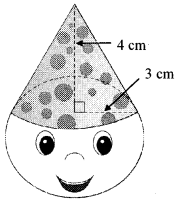Now, volume of the toy
= Volume of cone + volume of hemisphere
= 12π + 18π
= 30π
= 30 × 3.14
= 94.20 cm3
Also, surface area of the toy
= Curved surface area of cone + Curved surface area of hemisphere
= 15π + 18π
= 33π
= 33 × 3.14
= 103.62 cm2
∴ The volume and surface area of the toy are 94.20 cm3 and 103.62 cm2 respectively.

Question 11.
Find the surface area and the volume of a beach ball shown in the figure.Given: For the spherical ball,
diameter (d) = 42 cm
To find: Surface area and volume of the beach ball.
Solution:
Radius (r) = $$\frac { d }{ 2 }$$ = $$\frac { 42 }{ 2 }$$ = 21 cm
Surface area of sphere= 4πr2
= 4 × 3.14 × (21)2
= 4 × 3.14 × 21 × 21
= 5538.96 cm2
Volume of sphere = $$\frac { 4 }{ 3 }$$ πr3
= $$\frac { 4 }{ 3 }$$ × 3.14 × (21)3
= 4 × 3.14 × 7 × 21 × 21
= 38772.72 cm3
∴ The surface area and the volume of the beach ball are 5538.96 cm2 and 38772.72 cm3 respectively.

Question 12.
As shown in the figure, a cylindrical glass contains water. A metal sphere of diameter 2 cm is immersed in it. Find the volume of the water.Given: For the metal sphere,
diameter (d) = 2 cm
For the cylindrical glass, diameter (D) =14 cm,
height of water in the glass (H) = 30 cm
To find: Volume of water in the glass.
Solution:
Let the radii of the sphere and glass be r and R respectively.Volume of water with sphere in it = πR2H
= π × (7)2 × 30
= 1470π cm3
Volume of water in the glass
= Volume of water with sphere in it – Volume of sphere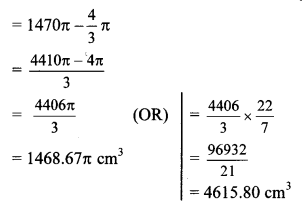∴ The volume of the water in the glass is 1468.67 π cm3 (i.e. 4615.80 cm3).

Maharashtra Board Class 10 Maths Chapter 7 Mensuration Intext Questions and Activities

Question 1.
The length, breadth and height of an oil can are 20 cm, 20 cm and 30 cm respectively as shown in the adjacent figure. How much oil will it contain? (1 litre = 1000 cm3) (Textbook pg. no.141)Given: For the cuboidal can,
length (l) = 20 cm,
height (h) = 30 cm
To find: Oil that can be contained in the can.
Solution:
Volume of cuboid = l × b × h
= 20 × 20 × 30
= 12000 cm3
= $$\frac { 12000 }{ 1000 }$$ litres
= 12 litres
∴ The oil can will contain 12 litres of oil.

Question 2.
The adjoining figure shows the measures of a Joker’s dap. How much cloth is needed to make such a cap? (Textbook pg. no. 141)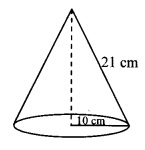Given: For the conical cap,
slant height (l) = 21 cm
To find: Cloth required to make the cap.
Solution:
Cloth required to make the cap
= Curved surface area of the conical cap
= πrl = $$\frac { 22 }{ 7 }$$ × 10 × 21
=22 × 10 × 3
= 660 cm2
∴ 660 cm2 of cloth will be required to make the cap.

Question 3.
As shown in the adjacent figure, a sphere is placed in a cylinder. It touches the top, bottom and the curved surface of the cylinder. If radius of the base of the cylinder is ‘r’,i. what is the ratio of the radii of the sphere and the cylinder ?
ii. what is the ratio of the curved surface area of the cylinder and the surface area of the sphere?
iii. what is the ratio of the volumes of the cylinder and the sphere? (Textbook pg. no. 141)
Solution:
∴ Radius of sphere = r
Also, height of cylinder = diameter of sphere
∴ h = d
∴ h = 2r …(i)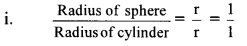∴ radius of sphere : radius of cylinder = 1 : 1.∴ curved surface area of cylinder : surface area of sphere = 1:1.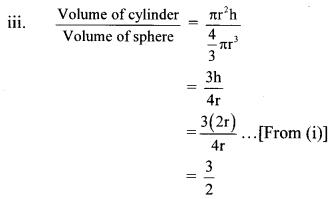∴ volume of cylinder : volume of sphere = 3 : 2.

Question 4.
Finding volume of a sphere using cylindrical beaker and water. (Textbook, pg. no. 142)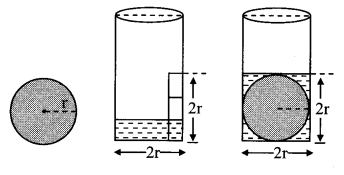i. Take a ball and a beaker of the same radius.
ii. Cut a strip of paper of length equal to the diameter of the beaker.
iii. Draw two lines on the strip dividing it into three equal parts.
iv. Stick this strip on the beaker straight up from the bottom.
v. Fill the water in the beaker upto the first mark of the strip from bottom.
vi. Push the ball in the beaker so that it touches the bottom.
Observe how much water level rises.
You will notice that the water level has risen exactly upto the total height of the strip. Try to obtain the formula for volume of sphere using the volume of the cylindrical beaker.
Solution:
Suppose volume of beaker upto height 2r is V.
V = πr2 h
∴ V = πr2(2r) …[∵ h = 2r]
∴ V = 2πr3
But, V = volume of the ball + volume of water in the beaker
∴ 2πr3 = Volume of the ball + $$\frac { 1 }{ 3 }$$ × 2πr3
∴ Volume of the ball = 2πr3 – $$\frac { 2 }{ 3 }$$ πr3
= $$\frac{6 \pi r^{3}-2 \pi r^{3}}{3}$$
∴ Volume of the ball = $$\frac { 4 }{ 3 }$$ πr3
∴ Volume of a sphere = $$\frac { 4 }{ 3 }$$ πr3